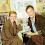## Sunday, September 10, 2017

### Statistics Sunday: What Are Degrees of Freedom? (Part 1)

I've had a few requests to write a post on degrees of freedom, an essential but not always well understood concept in statistics. Degrees of freedom are used in many statistical tests; they help you identify which row of a table you should use to find critical values and you probably had to calculate your degrees of freedom for multiple tests in introductory statistics. But there's rarely an explanation of what degrees of freedom are and why this concept is so important.

There are a couple ways you can think of degrees of freedom. For today's post, I'll present the typical way. But there's an additional way to understand degrees of freedom, that I'll write about next week. (I'm mostly splitting it up because of concerns about length, but I also find the idea of a cliffhanger statistics post quite entertaining! Edit: And now you don't even have to wait. You can find part 2 here.)

First up, the most literal definition of degrees of freedom - they are the number of values that are free to vary in calculating statistics. Let's say I tell you I have a sample of 100 people:

For each one, I have their score on the final exam of a traditional statistics course. Let's say I also tell you the mean of those 100 scores is 80.5. Can you recreate my sample based on that information alone?

You can't. Because there are many different configurations that could produce a mean of 80.5. It could be that half the sample had a score of 80 and the other half had a score of 81. And that's just one possibility.

So then I ask you, "How many scores do I need to give you before you can recreate my sample?"

The answer is 99. If you have 99 of the scores from the sample, you can figure out the last one, because that one is now determined. It can't be just any number; it has to be a number that results in a mean of 80.5 when combined with the 99 scores I gave you. That last value is no longer free to vary.

Let's keep going with that sample of test scores. I have 100 scores from people who took a basic statistics course. Now, let's say I have an additional 100 scores from people who took a combined statistics and methods course.

This is one of my pedagogical soap boxes: statistics should never be taught in isolation, but in the context of the type of research related to one's major. So I would hypothesize that my psychology majors who took a course that combined stats and methods will understand statistics better, and do better on the test, than students who took only statistics.

Full disclosure: My undergrad did a 2-semester combined stats and methods course with a lab component. I'm probably biased when I say that's how it should be done. I certainly wasn't an expert when I got out of that course, or even when I finished grad school; my current level of knowledge comes from years of practice, reading, and thinking. But I feel I had a much better understanding of statistics when I got to grad school than many of my classmates, so I had a solid foundation on which to build.

We'll keep the mean for the traditional stats course as 80.5. For the stats + methods group, let's say their mean is 87.5. I would compare these two means with each other using a t-test. But first, let's figure out our degrees of freedom. You already know that the degrees of freedom for the traditional course group is 99. How many degrees of freedom do we have for the stats + methods group? Also 99. All but that last score I give you is free to vary. So that gives us a total degrees of freedom of 198.

"But wait!" you say. "When I took introductory statistics, there was a formula to determine degrees of freedom for a t-test." And you would be right. That formula is N-2. I have 100 in each group, for a total of 200, and 200-2 would be 198. You'll find for many statistics involving 2 group comparisons (t-test, correlation coefficient), the degrees of freedom would be N-2. And that's because 99 of the scores in each group are free to vary.

But there's another way, a more conceptual way that gets at why degrees of freedom is important. That way of thinking becomes very helpful when determining degrees of freedom for ANOVA. Tune in next week for the exciting conclusion!

#### 1 comment:

1.Hi, Thankyou for this it was very helpful. Can you please explain why sometimes the df reported in analysis are some times less than N=2 (in two group comparison)? Is it due to missing values in the data? And in that case what does it mean for your data?